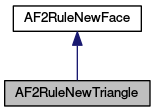MeshKit  1.0
AF2RuleNewTriangle Class Reference

`#include <AF2RuleNewTriangle.hpp>`

Inheritance diagram for AF2RuleNewTriangle:[legend]

List of all members.

## Public Member Functions

AF2RuleNewTriangle (unsigned int firstIndex, unsigned int secondIndex, unsigned int thirdIndex)
Constructor.
unsigned int getNumVertices () const
Get the number of vertices that the element this rule would create would have.
unsigned int getVertexIndex (unsigned int vtxNum) const
Get the index of one of the vertices of the element that the rule would create.

## Private Attributes

unsigned int triVtxIndices 

## Detailed Description

Definition at line 12 of file AF2RuleNewTriangle.hpp.

## Constructor & Destructor Documentation

 AF2RuleNewTriangle ( unsigned int firstIndex, unsigned int secondIndex, unsigned int thirdIndex )

Constructor.

The constructor requires arguments for the indices of the three vertices of the triangle that the rule would create. The indices must index into the list of vertices associated with whichever rules this AF2RuleNewTriangle is a member of. The list of vertices associated with the rule is the concatenation of the list of existing vertices in the rule and the list of new vertices that the rule would create.

Parameters:
 firstIndex the index of a vertex of the triangle secondIndex the index of the next vertex of the triangle after the firstIndex traversing the vertices counterclockwise thirdIndex the index of the remaining vertex of the element

Definition at line 6 of file AF2RuleNewTriangle.cpp.

## Member Function Documentation

 unsigned int getNumVertices ( ) const` [virtual]`

Get the number of vertices that the element this rule would create would have.

Returns:
the number of vertices that the element would have

Implements AF2RuleNewFace.

Definition at line 14 of file AF2RuleNewTriangle.cpp.

 unsigned int getVertexIndex ( unsigned int vtxNum ) const` [virtual]`

Get the index of one of the vertices of the element that the rule would create.

Indices returned by this method index into the list of vertices associated with the rule, i.e., a list consisting of a list of the rule's existing vertices followed by a list of the new vertices that the rule would create. The numbering of the vertices of the face begins with 0, so the valid arguments to this method are 0 through n - 1, where n is the value returned from getNumVertices().

Parameters:
 vtxNum the number of the vertex for which to get the index
Returns:
the index in the list of the rule vertices of vertex number vtxNum of the element

Implements AF2RuleNewFace.

Definition at line 19 of file AF2RuleNewTriangle.cpp.

## Member Data Documentation

 unsigned int triVtxIndices` [private]`

Definition at line 16 of file AF2RuleNewTriangle.hpp.

The documentation for this class was generated from the following files: